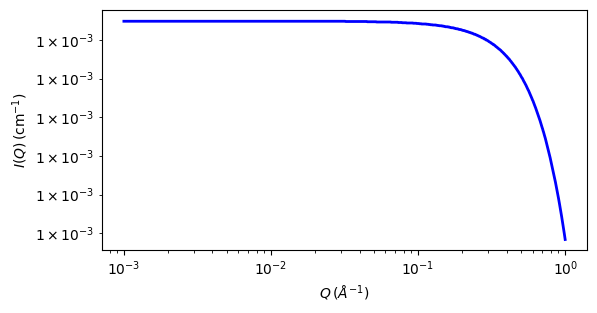# rpa

Random Phase Approximation

Parameter

Description

Units

Default value

scale

Scale factor or Volume fraction

None

1

background

Source background

cm-1

0.001

case_num

Component organization

None

1

N

Degree of polymerization

None

1000

Phi

volume fraction

None

0.25

v

molar volume

mL/mol

100

L

scattering length

fm

10

b

segment length

5

K12

A:B interaction parameter

None

-0.0004

K13

A:C interaction parameter

None

-0.0004

K14

A:D interaction parameter

None

-0.0004

K23

B:C interaction parameter

None

-0.0004

K24

B:D interaction parameter

None

-0.0004

K34

C:D interaction parameter

None

-0.0004

The returned value is scaled to units of cm-1 sr-1, absolute scale.

Warning

This model is not functioning correctly in SasView and it appears it has not done so for some time. Whilst the problem is investigated, a workaround for Case 0 below (the most common use case) is to use the binary_blend model available on the Model Maketplace . For further information, please email help@sasview.org . The SasView Developers. February 2022.

Definition

Calculates the macroscopic scattering intensity for a multi-component homogeneous mixture of polymers using the Random Phase Approximation. This general formalism contains 10 specific cases

Case 0: C/D binary mixture of homopolymers

Case 1: C-D diblock copolymer

Case 2: B/C/D ternary mixture of homopolymers

Case 3: C/C-D mixture of a homopolymer B and a diblock copolymer C-D

Case 4: B-C-D triblock copolymer

Case 5: A/B/C/D quaternary mixture of homopolymers

Case 6: A/B/C-D mixture of two homopolymers A/B and a diblock C-D

Case 7: A/B-C-D mixture of a homopolymer A and a triblock B-C-D

Case 8: A-B/C-D mixture of two diblock copolymers A-B and C-D

Case 9: A-B-C-D tetra-block copolymer

Note

These case numbers are different from those in the NIST SANS package!

The models are based on the papers by Akcasu et al.  and by Hammouda  assuming the polymer follows Gaussian statistics such that $$R_g^2 = n b^2/6$$ where $$b$$ is the statistical segment length and $$n$$ is the number of statistical segment lengths. A nice tutorial on how these are constructed and implemented can be found in chapters 28, 31 and 34, and Part H, of Hammouda’s ‘SANS Toolbox’ .

In brief, the macroscopic cross sections are derived from the general forms for homopolymer scattering and the multiblock cross-terms while the inter, polymer cross terms are described in the usual way by the $$\chi$$ parameter.

USAGE NOTES:

• Only one case can be used at any one time.

• The RPA (mean field) formalism only applies only when the multicomponent polymer mixture is in the homogeneous mixed-phase region.

• Component D is assumed to be the “background” component (ie, all contrasts are calculated with respect to component D). So the scattering contrast for a C/D blend $$\rho_{C/D} = [\rho_C - \rho_D]$$2.

• Depending on which case is being used, the number of fitting parameters can vary.

Note

• In general the degrees of polymerization, the volume fractions, the molar volumes, and the neutron scattering lengths for each component are obtained from other methods and held fixed while The scale parameter should be held equal to unity.

• The variables are normally the segment lengths ($$b_a$$, $$b_b$$, etc.) and $$\chi$$ parameters ($$K_{ab}$$, $$K_{ac}$$, etc).Fig. 118 1D plot corresponding to the default parameters of the model.

Source

rpa.py $$\ \star\$$ rpa.c

References

1. A Z Akcasu, R Klein and B Hammouda, Macromolecules, 26 (1993) 4136

1. Hammouda, Advances in Polymer Science 106 (1993) 87

2. B. Hammouda, SANS Toolbox https://www.ncnr.nist.gov/staff/hammouda/the_sans_toolbox.pdf.

Authorship and Verification

• Author: Boualem Hammouda - NIST IGOR/DANSE Date: pre 2010

• Converted to sasmodels by: Paul Kienzle Date: July 18, 2016